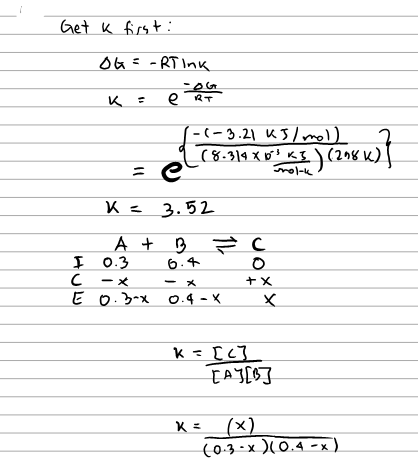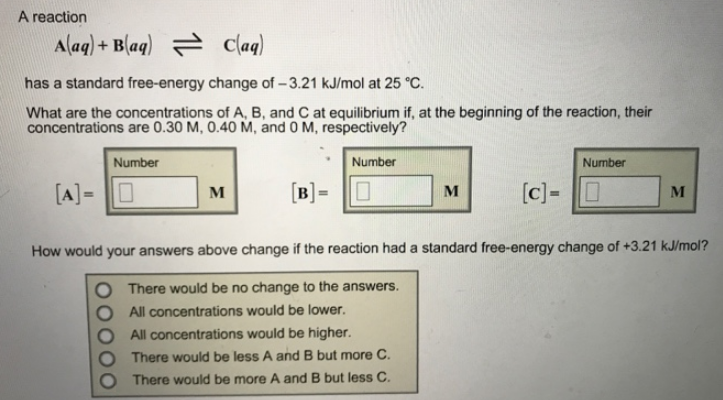# Problem: A reaction A (aq) + B (aq) ⇌ C (aq) has a standard free-energy change of -3.21 kJ/mol at 25°C. What are the concentrations of A, B, and C at equilibrium if, at the beginning of the reaction, their concentrations are 0.30 M, 0.40 M, and 0 M, respectively? How would your answers above change if the reaction had a standard free-energy change of +3.21 kJ/mol?                (a) There would be no change to the answers.                (b) All concentrations would be lower.                (c) All concentrations would be higher.                (d) There would be less A and B but more C.                (e) There would be more A and B but less C.

###### FREE Expert Solution80% (448 ratings)###### Problem Details

A reaction

A (aq) + B (aq) ⇌ C (aq)

has a standard free-energy change of -3.21 kJ/mol at 25°C.

What are the concentrations of A, B, and C at equilibrium if, at the beginning of the reaction, their concentrations are 0.30 M, 0.40 M, and 0 M, respectively?

How would your answers above change if the reaction had a standard free-energy change of +3.21 kJ/mol?

(a) There would be no change to the answers.

(b) All concentrations would be lower.

(c) All concentrations would be higher.

(d) There would be less A and B but more C.

(e) There would be more A and B but less C.